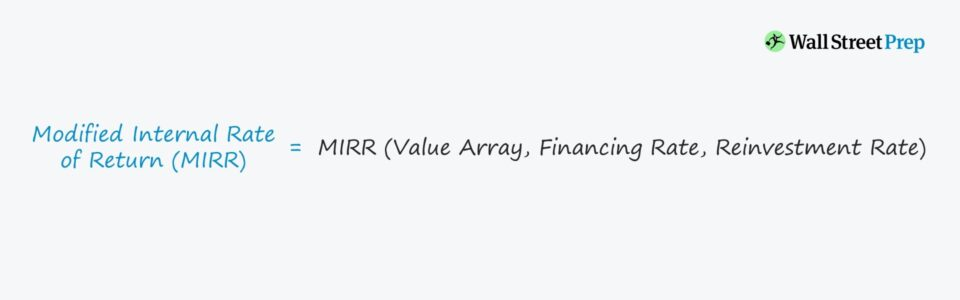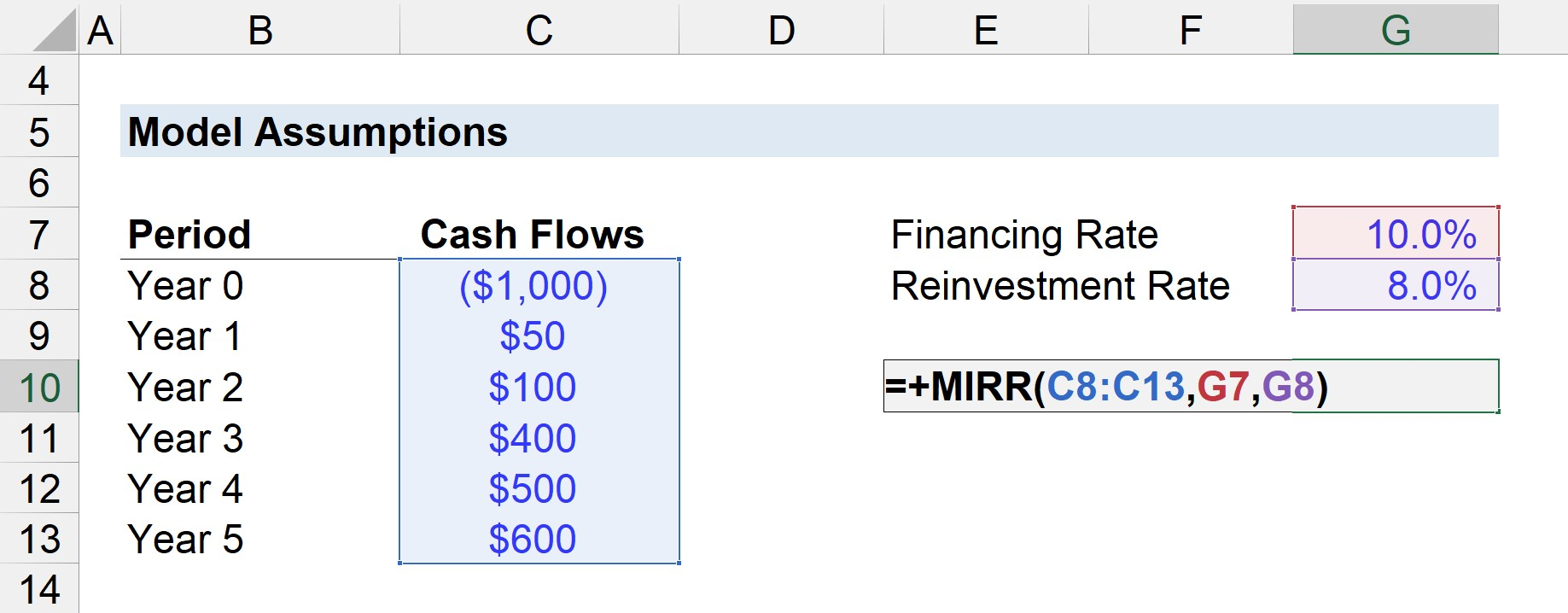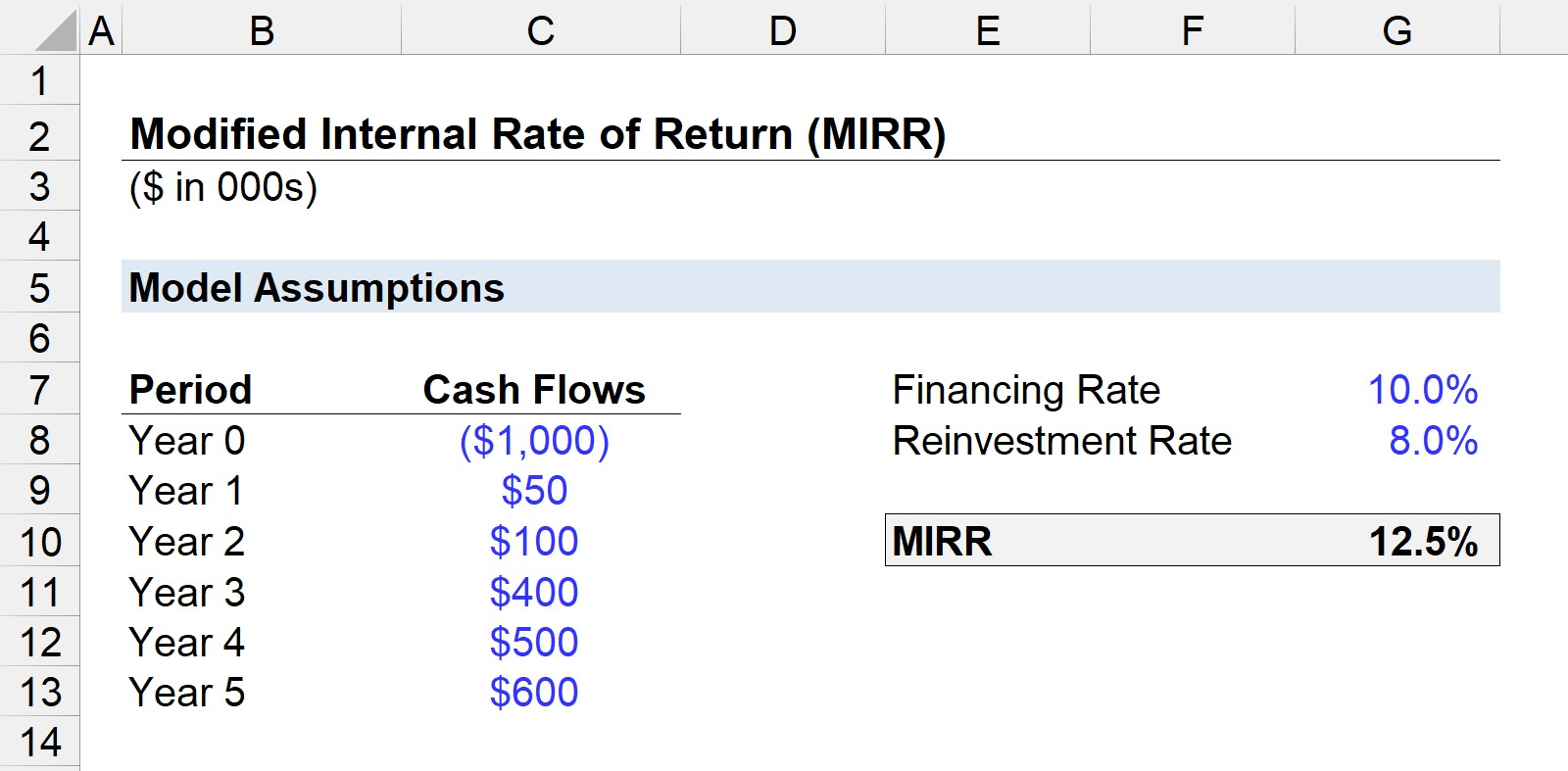# Modified Internal Rate of Return (MIRR)

Understand the MIRR Excel Function• What is the definition of the modified internal rate of return (MIRR)?
• How can the MIRR be calculated in Excel?
• What is the difference between the MIRR and IRR Excel function?
• How can MIRR be used for capital budgeting decisions?

## MIRR Excel Formula

MIRR stands for the “Modified Internal Rate of Return” and attempts to measure the potential profitability (and returns) from undertaking a project or investment.

As implied by the name, the MIRR Excel function is different from the traditional IRR function in that:

• Positive Cash Flows are Reinvested at the Reinvestment Rate
• Negative Cash Flows (i.e. the Initial Outlay) are Discounted at the Financing Rate

### MIRR Formula

The formula for calculating the MIRR is shown below.

• MIRR Excel Function = “MIRR(values, finance_rate, reinvest_rate)”

The inputs in the MIRR formula are as follows:

• values: The array or range of cells with the value of the cash flows, including the initial outflow.
• finance_rate: The cost of borrowing (i.e. interest rate) to fund the project or investment.
• reinvest_rate: The compounding rate of return at which positive cash flows are assumed to be reinvested.

Note that the initial outlay must be entered as a negative number in Excel.

## Interpreting MIRR

For purposes of capital budgeting, the following rules are generally followed:

• If MIRR > Cost of Capital ➝ Accept Project
• If MIRR < Cost of Capital ➝ Reject Project

When comparing numerous projects, the one with the highest MIRR is likely the one to pick, especially if other metrics also lead to the same conclusion.

## MIRR vs IRR Excel Function

The issue with the IRR Excel function is the implicit assumption that future positive cash flows are reinvested at the project or company’s cost of capital (i.e. required rate of return).

Critics of the IRR function argue that the assumption that the reinvestment rate is equal to the cost of capital overstates the return on the project or investment.

The reinvestment rate and cost of capital are often different in reality, so MIRR provides the option to specify a different reinvestment rate for future cash flows.

In effect, the MIRR Excel function is considered a more conservative measure compared to the IRR function (and usually results in a lower return).

## Drawbacks to MIRR

One limitation to the MIRR Excel function is that it assumes 100% of cash flows are reinvested into the project/company, which is not always the case.

Hypothetically, one could adjust the reinvestment rate and financing rate for each progressive stage, but doing so brings up the issue of uncertainty around the timing of specific actions.

In other words, attempting to precisely model out the reinvestment rate, financing rate, and cost of capital for each period does not necessarily add more accuracy to the analysis due to future uncertainty.

The IRR Excel function remains frequently used due to its simplicity, however.

## MIRR Excel Template

We can now move on to a modeling exercise to calculate the MIRR of a project.

To get started, fill out the form below to access the Excel file.Submitting ...

## MIRR Calculation Example

In our example scenario, we will be assuming a project has an initial cost of \$1 million.

After the initial cash outlay during the initial period (Year 0), the project is projected to produce the following cash flow amounts each year:

• Year 0: –\$1m
• Year 1: \$50k
• Year 2: \$100k
• Year 3: \$400k
• Year 4: \$500k
• Year 5: \$600k

As for the financing rate and reinvestment rate, we’ll assume the following:

• Financing Rate: 10%
• Reinvestment Rate: 12.5%

The greater the difference between the financing rate and reinvesting rate, the more that the IRR and MIRR will diverge from each other.

If we enter the provided assumptions into the MIRR formula, we get 12.5% as the MIRR.

The MIRR formula entered for our Excel model is shown below:Conversely, if we had used the IRR function, the resulting IRR is 14%, which demonstrates how MIRR is viewed as a more conservative measure.

But again, whether MIRR is more “accurate” or not is dependent on the amount of information on hand and the rationale behind the associated assumptions.Turbo-charge your time in Excel Used at top investment banks, Wall Street Prep's Excel Crash Course will turn you into an advanced Power User and set you apart from your peers.Inline FeedbacksLearn Excel Online

Become an Excel “Power User.” Used to train new hires at top financial institutions around the world!

X

The Wall Street Prep Quicklesson Series

7 Free Financial Modeling Lessons

Get instant access to video lessons taught by experienced investment bankers. Learn financial statement modeling, DCF, M&A, LBO, Comps and Excel shortcuts.